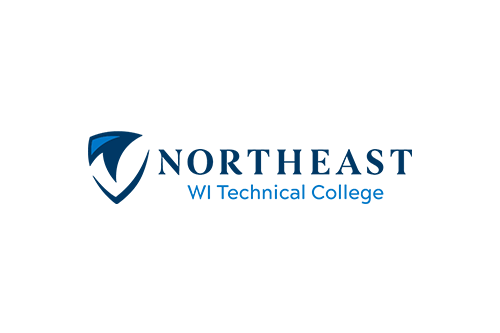# College Technical Math 1A

Course Number: 10804113
Credits: 3.00

## Course Description

10-804-113 COLLEGE TECHNICAL MATH 1A ...topics include solving linear equations; graphing; percent; proportions; measurement systems; computational geometry; and right triangle trigonometry. Emphasis will be on the application of skills to technical problems. (Prerequisite: Next Gen Arithmetic score greater/equal to 250 AND Rdg score greater/equal to 250; OR TABE A 9/10 Math greater/equal to 12.7 AND Rdg greater/equal to 8.8; OR TABE 11/12 Math greater/equal to 780 AND Rdg greater/equal to 573; OR ACT Math score greater/equal to 15 AND ACT Rdg score greater/equal to 16; OR preparatory courses-contact an academic advisor 920-498-5444)

• Summer
• Fall
• Spring

## Course Competencies

1. Perform basic operations with real numbers
2. Solve linear equations
3. Solve problems using percent and proportion
4. Solve variation problems
5. Graph algebraic functions
6. Relate the equation of a line to its graph
7. Convert units of measure
8. Compute angle measures, length of sides, perimeter, and area of plane geometric figures
9. Calculate surface area, volume, and weight/mass
10. Solve right triangles